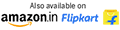||Call +91-11-43551180
Close

# Basic Mathematics

 Author Name : Kogent Learning Solutions Inc. Price INR : 269.00 ISBN 13 : 9789350045190 Release Date : 28 September 2012 With CD : No Pages : 212 Format : Paper Back Publisher : Dreamtech Press
Categories: , Product ID: 1912
Share

## Description

#### Book Description

The book Basic Mathematics provides easily understandable explanation of various concepts of mathematics. This book is unique, as it discusses about the essential concepts of mathematics, such as basic algebra and partial fractions, matrices and determinants, trigonometry, coordinate geometry, and measures of central tendency and dispersion. The book provides lots of examples in an easy-to-understand language along with exercises at the end of each unit.

In this book, you learn about:

• Polynomial fractions, partial fractions, resolving proper fraction into partial fraction, and resolving improper fraction into partial fraction
• Matrices, types of matrices, various operations on matrices, transpose and trace of a matrix, determinants and properties of determinants, minors and cofactors, and adjoint and inverse of a matrix
• Fundamental identities of trigonometry, trigonometric ratios of different angles, factorization formulas, defactorization formulas, and inverse trigonometric ratios
• Straight lines, distance formula, angle between two lines, parallel lines, and perpendicular lines
• Data analysis, measures of central tendency, and measures of dispersion

#### Table of ContentLoading...Taking too long?Reload document
|Open in new tab